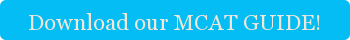The Cambridge Coaching Method of Solving Kinematics Questions™

How free body diagrams will figuratively save your life on the MCAT

Kinematics problems always take up a big chunk of time when I do 1 on 1 tutoring for the MCAT. They require practicing a methodical, rigorous approach if you’re going to do well on the test.  Today, I’d like to share with you the free body diagram tips that I’ve found are the most likely to ensure MCAT success.

Step 1: Take a guess about what you think is going to happen

This is an oft-neglected step. An MCAT question might start with something like “A 4 kilogram rock is dropped from a height of 10 m.” From that info alone, you know that the mass is going to move downwards and accelerate due to gravity. This is an important step because it both protects you from silly errors (I did the math and the rock floats up into space!) and also helps you build intuition for the problems. If something unexpected happens, you can adjust your intuition so that it’s stronger in the future.

Step 2: Choose axes (plural of axis, not what you chop wood with)

Every free body diagram should have a vertical and horizontal axis. Diagonal forces should be broken into components (if the angle is x degrees from horizontal, the vertical component of a diagonal force, F, at x degrees is Fsin(x) and the horizontal component is Fcos(x). Choose axes that make the math easier. For example, on an inclined plane, one axis should be perpendicular to the plane, and the other should be parallel to it.

Step 3: Account for all forces

Now, you need to start adding in the forces. There are generally six to seven forces you’ll have to deal with on the MCAT. They are:

1. Weight - typically calculated as mass times gravity
2. Normal force  - the force exerted when two surfaces are pushed against each other. The normal force is equal and opposite to the force pushing against the surface. So if weight is the only downward force, normal force is equal to weight.
3. Tension – the force exerted by a chord or wire pulling on an object; you’ll typically be given some data about this or have to deduce it.
4. Static friction- the force of friction when two surfaces are in contact and NOT sliding past each other. Note that rolling tires have static friction against the road, unless they are skidding, because the tire’s surface isn’t sliding past the road. Calculated as µS*(Normal Force). However, this is the MAXIMUM value of static friction. Static friction will act in an equal and opposite direction to all other forces, unless even maximum static fiction does not prevent movement. In that case you have….
5. Kinetic friction-The force of friction when two surfaces are in contact and sliding past each other. This is a resistive force, so it will always point in a direction opposite the motion. For example, a skier sliding down a mountain’s friction is pointing UP the mountain because the skier is moving DOWN the mountain.
6. Air resistance- You will often be told to ignore air resistance on the MCAT, but if you’re not, the basics are that it increases with increasing cross sectional area, and increasing velocity. Terminal velocity is a condition in which a falling object is moving so rapidly that the force of air resistance is equal to the force of weight, and the object is no longer accelerating.
7. Miscellaneous- these are things like the force of a spring, or a rocket, or your little brother pushing a wagon that the MCAT might throw at you. They will typically be described very clearly.

Step 4- Remember that Fnet=ma, and that if you have two, you can always solve for the third…

Now you have all the information you need to solve your MCAT problem. Simply take the mass and the force if you have it, and figure out the acceleration in the vertical or horizontal direction. OR take the mass and the acceleration, and figure out the force. Remember that the MCAT will give you clues like “at rest” to say that acceleration is 0. If you’re still having issues, consider reaching out to Cambridge Coaching for a free consultation with one of our MCAT tutors. We offer expert MCAT tutoring in New York, Boston, and online.

Happy free-body diagramming!